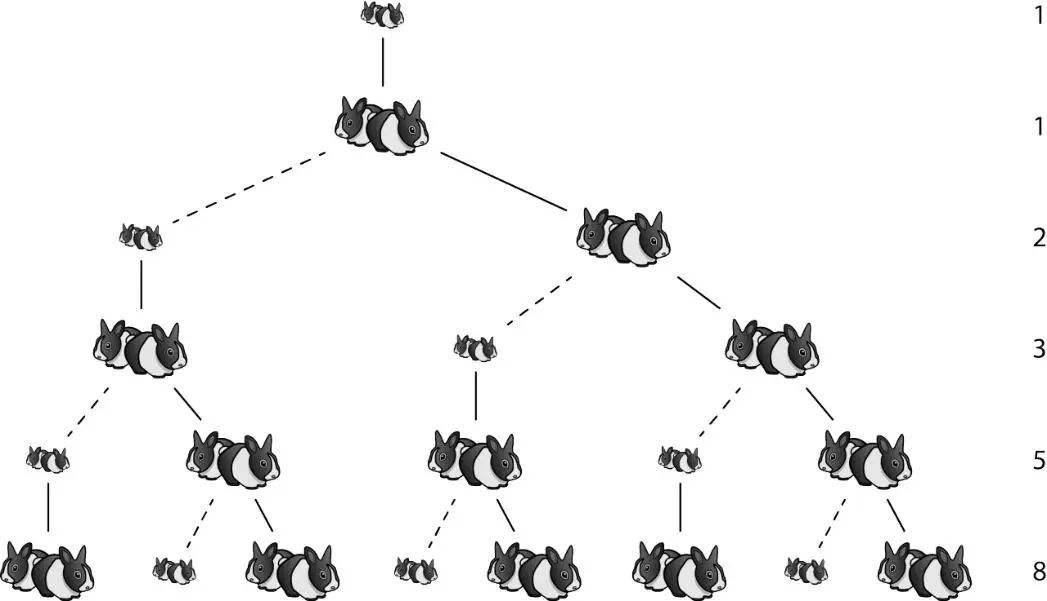## 性质

a.f(0)-f ⑴ +f ⑵ -…+(-1)^n·f(n)=(-1)^n·[f(n+1)-f(n)]-1。

b.f(n+m)=f(n+1)·f(m)+f(n)·f(m-1)。

c.f ⑴ +f ⑶ +f ⑸ +…+f(2n-1)=f(2n）。

d.f(0)+f ⑴ +f ⑵ +…+f(n)=f(n+2)-1。

e.f ⑵ +f ⑷ +f ⑹ +…+f(2n)=f(2n+1)-1。

f.[f(0)]^2+[f ⑴ ]^2+…+[f(n)]^2=f(n）·f(n+1）。

## 解法

``````/**
*  斐波那契数列问题
* 1.数组实现  1 1 2 3 5 8 13....
*   a=1,a=1;
*   a[x>=2]=a[x-1]+a[x-2];
*
* 2.变量变化实现    a   b
*                 1   1
*                 1   2
*                 2   3
*                 3   5
*                 5   8
*   a=1,b=1;
*   tem=a;
*   a=b;
*   b=b+tem;
* 3.递归实现
*/
public static int fib(int n) {
if (n == 0)
return 0;
if (n == 1 || n == 2) {
return n;
} else {
return fib(n - 1) + fib(n - 2);
}
}``````

x2=x+1解得：

## 实际应用

QCLDPC 码

QCLDPC 是一类半结构化的低密度奇偶校验码, 其分块的矩阵结构具有超大规模集成电路实现上的便利, 同时保持了优异的纠错性能。可基于斐波那契数列提出了一种准循环低密度奇偶校验码的新颖构造方法, 该构造方法能避免四环的产生, 具有较好的纠错性能, 可通过改变参数值进而改变码长和码率。对任意（m0,n0), 令m0<m1,n0<n1 有pm0,n1-pm0,n0<pm1,n1-pm1,n0,0<pm0,n1-pm0,n0<p,0<pm1,n1-pm1,n0<p由此可以推出（pm0,n1-pm0,n0）+（pm1,n1-pm1,n0） 不等于0modp。那么使用斐波那契数列构造的矩阵不含四线循环。

## 参考

1. ^曹艳华，吕广红.几种求广义斐波那契数列的 Matlab实现方法[J]• 大学教育，2016，（1):96 -97.
2. ^郑英元. 斐波那契数列[ J 1 数学教学，2009, (2)：49.
3. ^宋庭武．用特征方程推导斐波那契数列的通项公式［Ｊ］．安庆师范学院学报（自然科学版），２０１０，１６（４）：９１－９２
4. ^华东师范大学数学系．数学分析［Ｍ］．３版．北京：高等教育出版社，２００１：４７－６３
5. ^张新娟．斐波那契数列通项公式的求法［Ｊ］．高等数学研究，２００９，１２（４）：５６－５９
6. ^詹伟, 朱光喜, 彭立. 利用斐波那契数列构 造QCLDPC 码的方法[J]. 华中科技大学学报（ 自然科学 版）,2008,36(10):63-65.
7. ^吴振奎. 斐波那契数列欣赏[M]. 哈尔滨工业大学出 版社,2012. 神奇的黄金螺旋-《数学大王( 中高年级)》
8. ^贾菲菲. 斐波那契数列的研究与应用[J]. 科技创新与应 用,2014,(13):53.